# Measure RTD Sensors without a Precision Current Source

## Diodes DMMT3906W MMBT3904 MMBT3906

You can achieve accurate resistance-temperature-detector sensor measurements without having to resort to using a precision current source.

Traditionally, resistance-temperature-detector (RTD) sensor resistance is measured by applying a precision current source and measuring the developed voltage. This approach usually requires a precision voltage reference to create the current source, followed by a high-quality analog-to-digital converter (ADC) to measure the voltage.

This isn’t difficult to achieve at room temperature, but when you consider that the temperature of your measuring system can be in the range of −40 to +55 °C, the task becomes more daunting.

A brute-force approach to this problem would be to use an expensive temperature-stable voltage reference, ADC, and other components combined with software calibration to compensate for the temperature drift of parameters. This approach is complex and will fail to achieve the high precision that borderlines the sensor accuracy.

A better approach was discovered using 5 ppm/°C ultra-stable resistors with 0.1% accuracy as a reference for RTD measurements. This approach requires two onboard ultra-stable resistors for calibration (1 k and 2 k) to achieve high RTD precision. Those resistors are used to calibrate the RTD reading and compensate for temperature-drift errors.

The design uses the Q1-Q3 transistors in combination with the R1 resistor to form a constant-current source that sources about 1 mA using a 2.5-V ADC voltage reference (see Figure 1). Calibration resistors R4 and R5 along with the RT1 and RT2 RTD sensors can sink this current when the corresponding GPIO pin is driven low. When not used, the GPIO pins are tri-stated. The voltage is measured at the ADC output.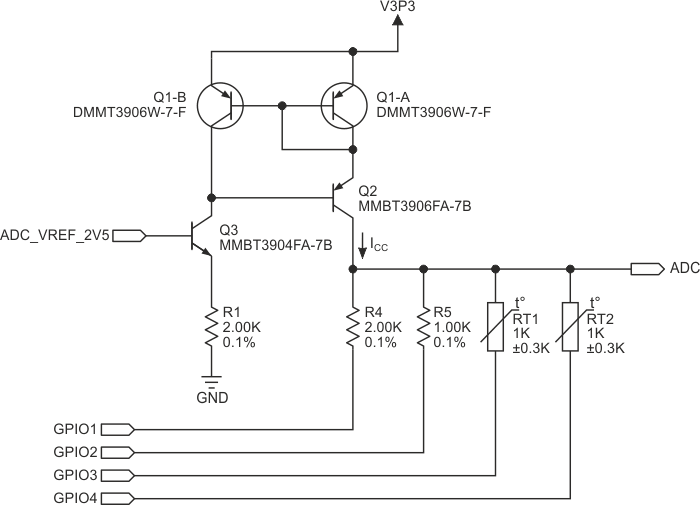Figure 1. The Q1-Q3 transistors and R1 resistor form a constant-current source that sources about 1 mA using a 2.5-V ADC voltage reference.

For calibration, we will need to read two resistors and calculate the constant-current source value and combined errors, which we call VOFFSET. The calibrated ICC and VOFFSET values are used to convert RTD temperature readings.

Table 1. The Q1-Q3 transistors and R1 resistor form a constant-current source that
sources about 1 mA using a 2.5-V ADC voltage reference.
 Sample VCAL1K VCAL2K RTD 1 RTD 2 Calibration Values ADC [V] ADC [V] ADC [V] R [Ohm] Temp [C°] ADC [V] R [Ohm] Temp [C°] VOFFSET [V] ICC [A] 1 1.208 2.353 1.350 1123.94 31.90 1.315 1092.96 23.90 0.06445 0.00114 2 1.208 2.353 1.347 1121.13 31.10 1.315 1092.96 23.90 0.06445 0.00114 3 1.196 2.349 1.331 1117.32 30.20 1.321 1108.94 28.00 0.04189 0.00115 4 1.196 2.349 1.350 1134.08 34.50 1.321 1108.94 28.00 0.04189 0.00115 5 1.196 2.349 1.334 1120.11 30.90 1.321 1108.94 28.00 0.04189 0.00115

The calibration results (see Table 1) are applied using following formula: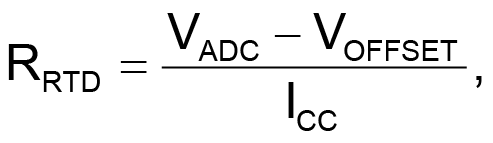(1)

where:

RRTD is the measured RTD resistance;
VADC is the ADC voltage reading;
ICC is a somewhat constant-current source;
VOFFSET is the voltage offset of cumulative errors.

Note that VOFFSET is a combination of multiple error voltage sources. Therefore, it might be beneficial (though not necessary) to split into its components to get better precision.

To calculate VOFFSET and ICC, we need to make a few assumptions to derive the formula below:

• First assumption: Calibration resistors are ideal and have 1,000- and 2,000-Ω values, respectively.
• Second assumption: The ICC current source is stable for the duration of measurements.
• Third assumption: ADC conversion results are perfect.

Following those assumptions, we can write that: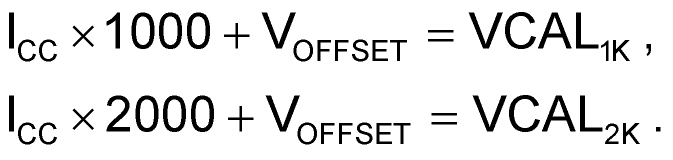(2)

In Equation 2, VCAL1K and VCAL2K represent voltages developed on calibration resistors when ICC current is applied.

By solving those equations for ICC and VOFFSET, we get the following equations: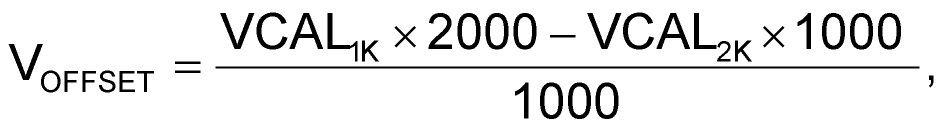(3)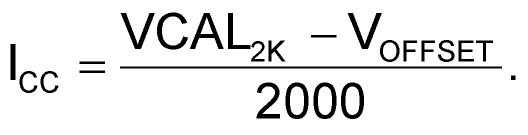(4)

## Experimental Setup Measurements

The experimental setup has two calibration resistors and two RTDs mounted on different locations. We used an ADC with 10-bit resolution and surface-mount RTDs rated 1 k at room temperature. Notice how the calibration values change when board temperature changes between sample 2 and 3 in the Table 1.

To gather the data, the software followed these steps:

1. Read ADC voltage levels on calibration resistors and RTDs.
2. Calculate VOFFSET using Equation 3.
3. Calculate ICC with Equation 4.
4. Determine RTD resistance utilizing Equation 1.
5. Convert RTD resistance values to temperature with the table lookup and piecewise interpolation.

## Materials on the topic

electronicdesign.comYou may have to register before you can post comments and get full access to forum.
 User Name Remember Me? Password